# Ages

Father is 36 years old, his daughter is 4 years old. Write down the ratio of the age of father and daughter. In what ratio will the ages of father and daughter after 4 years?

Correct result:

x =  9:1
y =  5:1

#### Solution:

$y=\frac{f+4}{d+4}=\frac{36+4}{4+4}=5=5:1$We would be pleased if you find an error in the word problem, spelling mistakes, or inaccuracies and send it to us. Thank you!Tips to related online calculators
Need help calculate sum, simplify or multiply fractions? Try our fraction calculator.
Check out our ratio calculator.
Do you want to convert time units like minutes to seconds?

## Next similar math problems:

• Father and daughterFather is 36 years old, daughter is 20 years less. What will be the ratio between them when they are 10 years more?
• Family and age ratiosAge of father and son are in the ratio 10:3; age of father and daughter are in the ratio 5:2. How old is father and son, if daughter is 20 years old? In what ratio is age sister and brother?
• Age of familyThe age of father and son is in the ratio 10: 3. The age of the father and daughter is in ratio 5: 2. How old are a father and a son if the daughter is 20?
• Honza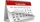Honza is 13 years old and Peter 21 years old. After how many years are their ages will be at ratio 7: 9?
• Father 7Father is 6 times older than his son. After 4 years, the father will only be 4 times older. What are their present ages?
• Age ratioJanko is 14 years old. The age ratio of Janka and Zuzka is 2: 3. What was the ratio seven years ago?
• Ages 2A man's age is 4 times his son's age. After 5 years he will be just twice his son's age, find their ages.
• Jan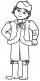Jan is 13 years and Peter 21 years old. For how many years will their ages in the ratio of 7:9?
• Age problemsA) Alex is 3 times as old as he was 2 years ago. How old is he now? b) Casey was twice as old as his sister 3 years ago. Now he is 5 years older than his sister. How old is Casey? c) Jessica is 4 years younger than Jennifer now. In 10 years, Jessica will
• Family 8Father is 38 years old, daughter 12, son 14. How many years will father have as many years as his children together?
• The agesThe ages of the four sons make an arithmetic sequence, the sum of which is the age of the father today. In three years, the father's age will be the sum of the ages of the three eldest sons, and in the next two years and three months, the father's age wil
• Mother and daughter 2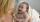The mother is 40 years older than her daughter. How old is the mother if her age is eight thirds age of daughter?
• SuzanSusan's age will be after 12 years four times as much as twelve years ago. How old is Susan now?
• Eight years agoThe son asks his father how old he is. My father said: In ten years, I will have twice as much as I had eight years ago.
• Mother and daughter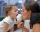Three years ago mother was three times older than daughter. After nine years she will be only twice old. How old is mother (and daughter)?
• FamilyMom is 42 years old and her daughters 13 and 19. After how many years will mother as old as her daughter together?
• Father and sons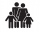Father is 27 and his sons 2 and one year. In how many years will his sons sum up to half his age?